## [Technology Sharing] Logistic regression classification

Author: After Yin Di, authorized release.

## 1. Binary Logistic Regression

Regression is an easy-to-understand model, which is equivalent to `y=f(x)`indicating the relationship between independent `x`and dependent variables `y`. The most common problems are the doctor's hope, smell, ask, and cut during treatment, and then determine whether the patient is sick or what kind of disease, among which the look, smell, ask, and cut are the independent variables obtained `x`, that is, the characteristic data, to judge whether they are sick It is equivalent to obtaining the dependent variable `y`, that is, the predicted classification. The simplest regression is linear regression, but the robustness of linear regression is poor.

Logistic regression is a `[0,1]`regression model that reduces the prediction range and limits the prediction value to time. The regression equation and regression curve are shown in the following figure. In the logistic curve `z=0`, the sensitive, at `z>>0`or `z<<0`when not sensitive.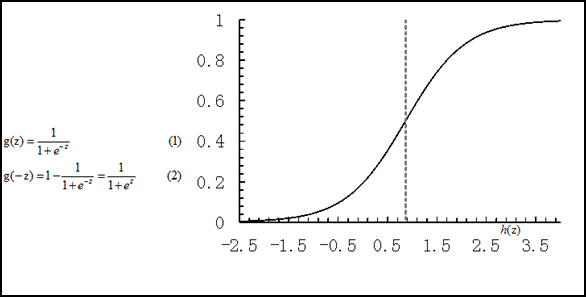Logistic regression is actually based on linear regression, applying a logical function. The picture above shows `g(z)`this logical function (or `Sigmoid`function). The left picture below is a linear decision boundary, and the right picture is a nonlinear decision boundary.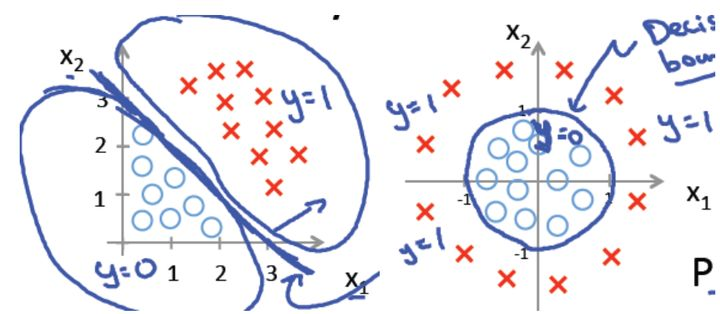For the case of linear boundaries, the boundary form can be summarized as the following formula  (1) :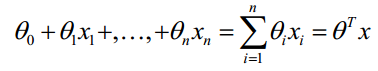Therefore, we can construct the prediction function as the following formula  (2) :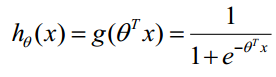The prediction function represents the probability when the classification result is 1. Therefore, for the input points `x`, the probability that the classification results are category 1 and category 0 are as follows  (3) :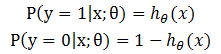For the training data set, feature data `x={x1, x2, … , xm}`and corresponding classification data `y={y1, y2, … , ym}`. To construct a logistic regression model `f`, the most typical construction method is to apply maximum likelihood estimation.  Taking the maximum likelihood function for formula  (3) , the following formula (4) can be obtained  :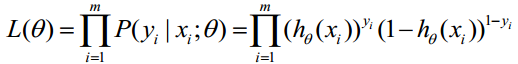Then  take the logarithm of formula  (4) to get formula  (5) :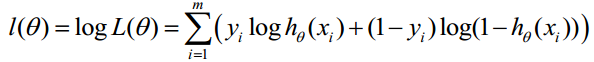The maximum likelihood estimation is to `l`obtain the maximum value `theta`. `MLlib`There are two methods to find this parameter, namely gradient descent and L-BFGS.

## 2. Multiple logistic regression

Binary logistic regression can be generalized to multiple logistic regression to train and predict multiple classification problems. For multi-classification problems, the algorithm will train a multiple logistic regression model, which contains `K-1`a binary regression model. Given a data point, `K-1`all models will run, and the category with the highest probability will be selected as the prediction category.

For the input points `x`, the probability that the classification result is each category is as follows  (6)  , which `k`represents the number of categories.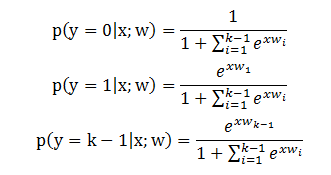For `k`multi-class classification problems, the weight `w = (w_1, w_2, ..., w_{K-1})`of the model is a matrix. If the intercept is added, the dimension of the matrix is `(K-1) * (N+1)`, otherwise `(K-1) * N`. The loss function of the objective function of a single sample can be written as the following formula  (7)  .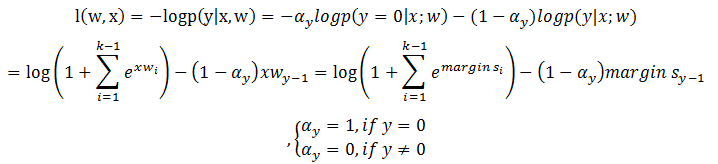Finding the first derivative of the loss function, we can get the following formula  (8) :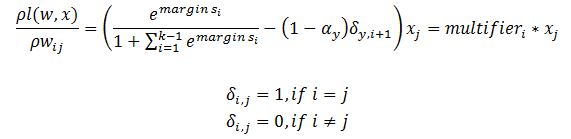According to the above formula, if some `margin`value is greater than 709.78, `multiplier`and the calculation of the logic function will occur arithmetic overflow ( `arithmetic overflow`). This problem occurs when there are outliers far away from the hyperplane. Fortunately, when `max(margins) = maxMargin > 0`, the loss function can be rewritten as the following equation  (9)  form.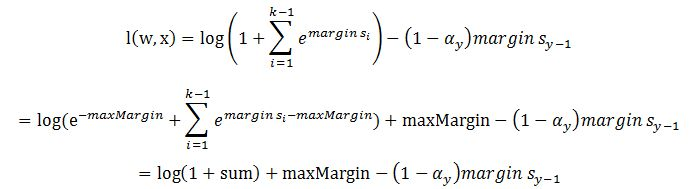Similarly, `multiplier`it can also be rewritten as the following formula  (10)  .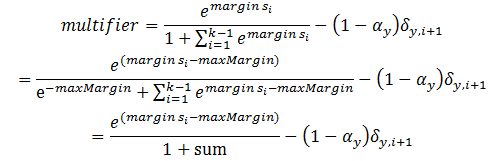• Advantages: low computational cost, fast speed, easy to understand and implement.
• Disadvantages: easy to underfit, and the accuracy of classification and regression is not high.

## 4. Examples

The following example shows how to use logistic regression.

``````import org.apache.spark.SparkContext
import org.apache.spark.mllib.classification.{LogisticRegressionWithLBFGS, LogisticRegressionModel}
import org.apache.spark.mllib.evaluation.MulticlassMetrics
import org.apache.spark.mllib.regression.LabeledPoint
import org.apache.spark.mllib.linalg.Vectors
import org.apache.spark.mllib.util.MLUtils
// 加载训练数据
// 切分数据，training (60%) and test (40%).
val splits = data.randomSplit(Array(0.6, 0.4), seed = 11L)
val training = splits(0).cache()
val test = splits(1)
// 训练模型
val model = new LogisticRegressionWithLBFGS()
.setNumClasses(10)
.run(training)
// Compute raw scores on the test set.
val predictionAndLabels = test.map { case LabeledPoint(label, features) =>
val prediction = model.predict(features)
(prediction, label)
}
// Get evaluation metrics.
val metrics = new MulticlassMetrics(predictionAndLabels)
val precision = metrics.precision
println("Precision = " + precision)
// 保存和加载模型
model.save(sc, "myModelPath")

## 5 Source code analysis

### 5.1 Training the model

As mentioned above, in `MLlib`, gradient descent method and `L-BFGS`logistic regression parameter calculation are used respectively . We will introduce the implementation of these two algorithms in the optimization section, here we introduce the public part.

`LogisticRegressionWithLBFGS`And the `LogisticRegressionWithSGD`entry function are `GeneralizedLinearAlgorithm.run`the following detailed analysis of the process.

``````def run(input: RDD[LabeledPoint]): M = {
if (numFeatures < 0) {
//计算特征数
numFeatures = input.map(_.features.size).first()
}
val initialWeights = {
if (numOfLinearPredictor == 1) {
Vectors.zeros(numFeatures)
Vectors.zeros((numFeatures + 1) * numOfLinearPredictor)
} else {
Vectors.zeros(numFeatures * numOfLinearPredictor)
}
}
run(input, initialWeights)
}``````

The above code initializes the weight vector, and the value of the vector is initialized to 0. It should be noted `addIntercept`that whether to add the intercept ( `Intercept`, refers to the distance between the intersection point of the function graph and the coordinate to the origin), the default is not added. `numOfLinearPredictor`Represents the number of binary logistic regression models. We focus on `run(input, initialWeights)`the realization. It is implemented in four steps.

### 5.1.1 Scaling features and adding intercepts according to the provided parameters

``````val scaler = if (useFeatureScaling) {
new StandardScaler(withStd = true, withMean = false).fit(input.map(_.features))
} else {
null
}
val data =
if (useFeatureScaling) {
input.map(lp => (lp.label, appendBias(scaler.transform(lp.features)))).cache()
} else {
input.map(lp => (lp.label, appendBias(lp.features))).cache()
}
} else {
if (useFeatureScaling) {
input.map(lp => (lp.label, scaler.transform(lp.features))).cache()
} else {
input.map(lp => (lp.label, lp.features))
}
}
val initialWeightsWithIntercept = if (addIntercept && numOfLinearPredictor == 1) {
appendBias(initialWeights)
} else {
/** If `numOfLinearPredictor > 1`, initialWeights already contains intercepts. */
initialWeights
}``````

In the optimization process, the speed of convergence depends on the number of conditions ( `condition number`) in the training data set , and scaling variables can often heuristically reduce these number of conditions and increase the speed of convergence. Without reducing the number of conditions, some data sets mixed with columns of different ranges may not converge. Here `StandardScaler`, the features of the data set are used for scaling. `appendBias`The method is very simple, is to add an item with a value of 1 after each vector.

``````def appendBias(vector: Vector): Vector = {
vector match {
case dv: DenseVector =>
val inputValues = dv.values
val inputLength = inputValues.length
val outputValues = Array.ofDim[Double](inputLength + 1)
System.arraycopy(inputValues, 0, outputValues, 0, inputLength)
outputValues(inputLength) = 1.0
Vectors.dense(outputValues)
case sv: SparseVector =>
val inputValues = sv.values
val inputIndices = sv.indices
val inputValuesLength = inputValues.length
val dim = sv.size
val outputValues = Array.ofDim[Double](inputValuesLength + 1)
val outputIndices = Array.ofDim[Int](inputValuesLength + 1)
System.arraycopy(inputValues, 0, outputValues, 0, inputValuesLength)
System.arraycopy(inputIndices, 0, outputIndices, 0, inputValuesLength)
outputValues(inputValuesLength) = 1.0
outputIndices(inputValuesLength) = dim
Vectors.sparse(dim + 1, outputIndices, outputValues)
case _ => throw new IllegalArgumentException(s"Do not support vector type \${vector.getClass}")
}``````

### 5.1.2 Use the optimization algorithm to calculate the final weight value

``val weightsWithIntercept = optimizer.optimize(data, initialWeightsWithIntercept)``

There are gradient descent algorithm and `L-BFGS`two algorithms to calculate the final weight value. `Gradient`The implementation classes used by both algorithms calculate gradients, and `Updater`the implementation classes used update parameters. In  `LogisticRegressionWithSGD` and  `LogisticRegressionWithLBFGS` , they both use the  `LogisticGradient` implementation class to calculate the gradient and the  `SquaredL2Updater` implementation class to update the parameters.

``````//在GradientDescent中
private val updater = new SquaredL2Updater()
.setStepSize(stepSize)
.setNumIterations(numIterations)
.setRegParam(regParam)
.setMiniBatchFraction(miniBatchFraction)
//在LBFGS中
override val optimizer = new LBFGS(new LogisticGradient, new SquaredL2Updater)``````

The `LogisticGradient`implementation and `SquaredL2Updater`implementation will be described in detail below .

`LogisticGradient`Use the `compute`method to calculate the gradient. The calculation is divided into two cases, namely the case of binary logistic regression and the case of multiple logistic regression. Although multiple logistic regression can also achieve binary classification, for efficiency, the `compute`method still implements a version of binary logistic regression.

``````val margin = -1.0 * dot(data, weights)
val multiplier = (1.0 / (1.0 + math.exp(margin))) - label
//y += a * x，即cumGradient += multiplier * data
if (label > 0) {
// The following is equivalent to log(1 + exp(margin)) but more numerically stable.
MLUtils.log1pExp(margin)
} else {
MLUtils.log1pExp(margin) - margin
}``````

Here `multiplier`is the formula (2) above   . `axpy`The method is used to calculate the gradient, which means here `h(x) * x`. The following is the realization method of multiple logistic regression.

``````//权重
val weightsArray = weights match {
case dv: DenseVector => dv.values
case _ =>
throw new IllegalArgumentException
}
//梯度
case dv: DenseVector => dv.values
case _ =>
throw new IllegalArgumentException
}
// 计算所有类别中最大的margin
var marginY = 0.0
var maxMargin = Double.NegativeInfinity
var maxMarginIndex = 0
val margins = Array.tabulate(numClasses - 1) { i =>
var margin = 0.0
data.foreachActive { (index, value) =>
if (value != 0.0) margin += value * weightsArray((i * dataSize) + index)
}
if (i == label.toInt - 1) marginY = margin
if (margin > maxMargin) {
maxMargin = margin
maxMarginIndex = i
}
margin
}
//计算sum，保证每个margin都小于0，避免出现算术溢出的情况
val sum = {
var temp = 0.0
if (maxMargin > 0) {
for (i <- 0 until numClasses - 1) {
margins(i) -= maxMargin
if (i == maxMarginIndex) {
temp += math.exp(-maxMargin)
} else {
temp += math.exp(margins(i))
}
}
} else {
for (i <- 0 until numClasses - 1) {
temp += math.exp(margins(i))
}
}
temp
}
//计算multiplier并计算梯度
for (i <- 0 until numClasses - 1) {
val multiplier = math.exp(margins(i)) / (sum + 1.0) - {
if (label != 0.0 && label == i + 1) 1.0 else 0.0
}
data.foreachActive { (index, value) =>
if (value != 0.0) cumGradientArray(i * dataSize + index) += multiplier * value
}
}
//计算损失函数,
val loss = if (label > 0.0) math.log1p(sum) - marginY else math.log1p(sum)
if (maxMargin > 0) {
loss + maxMargin
} else {
loss
}``````
• SquaredL2Updater
``````class SquaredL2Updater extends Updater {
override def compute(
weightsOld: Vector,
stepSize: Double,
iter: Int,
regParam: Double): (Vector, Double) = {
// w' = w - thisIterStepSize * (gradient + regParam * w)
// w' = (1 - thisIterStepSize * regParam) * w - thisIterStepSize * gradient
//表示步长，即负梯度方向的大小
val thisIterStepSize = stepSize / math.sqrt(iter)
val brzWeights: BV[Double] = weightsOld.toBreeze.toDenseVector
//正则化，brzWeights每行数据均乘以(1.0 - thisIterStepSize * regParam)
brzWeights :*= (1.0 - thisIterStepSize * regParam)
//y += x * a，即brzWeights -= gradient * thisInterStepSize
//正则化||w||_2
val norm = brzNorm(brzWeights, 2.0)
(Vectors.fromBreeze(brzWeights), 0.5 * regParam * norm * norm)
}
}``````

The implementation rules of this function are:

``````w' = w - thisIterStepSize * (gradient + regParam * w)
w' = (1 - thisIterStepSize * regParam) * w - thisIterStepSize * gradient``````

This `thisIterStepSize`indicates the rate at which the parameter changes in the direction of the negative gradient, which decreases as the number of iterations increases.

### 5.1.3 Post-processing the final weight value

``````val intercept = if (addIntercept && numOfLinearPredictor == 1) {
weightsWithIntercept(weightsWithIntercept.size - 1)
} else {
0.0
}
var weights = if (addIntercept && numOfLinearPredictor == 1) {
Vectors.dense(weightsWithIntercept.toArray.slice(0, weightsWithIntercept.size - 1))
} else {
weightsWithIntercept
}``````

This code obtained the intercept ( `intercept`) and the final weight value. Since the intercept ( `intercept`) and weights are trained in a contracted space, we need to convert them to the original space again. Mathematical knowledge tells us that if we only perform normalization without subtracting the mean, that is `withStd = true, withMean = false`, `intercept`the value of the intercept ( ) will not send changes. So the following code only deals with weight vectors.

``````if (useFeatureScaling) {
if (numOfLinearPredictor == 1) {
weights = scaler.transform(weights)
} else {
var i = 0
val n = weights.size / numOfLinearPredictor
val weightsArray = weights.toArray
while (i < numOfLinearPredictor) {
//排除intercept
val start = i * n
val end = (i + 1) * n - { if (addIntercept) 1 else 0 }
val partialWeightsArray = scaler.transform(
Vectors.dense(weightsArray.slice(start, end))).toArray
System.arraycopy(partialWeightsArray, 0, weightsArray, start, partialWeightsArray.size)
i += 1
}
weights = Vectors.dense(weightsArray)
}
}``````

### 5.1.4 Create a model

``createModel(weights, intercept)``

### 5.2 Forecast

After training the model, we can calculate the classification information of the test data through the trained model. `predictPoint`Used to predict classification information. It deals with binary classification and multi-classification separately.

• The second classification
``````val margin = dot(weightMatrix, dataMatrix) + intercept
val score = 1.0 / (1.0 + math.exp(-margin))
threshold match {
case Some(t) => if (score > t) 1.0 else 0.0
case None => score
}``````

We can see `1.0 / (1.0 + math.exp(-margin))`that the above mentioned logical function is the `sigmoid`function.

• Multi-classification
``````var bestClass = 0
var maxMargin = 0.0
val withBias = dataMatrix.size + 1 == dataWithBiasSize
(0 until numClasses - 1).foreach { i =>
var margin = 0.0
dataMatrix.foreachActive { (index, value) =>
if (value != 0.0) margin += value * weightsArray((i * dataWithBiasSize) + index)
}
// Intercept is required to be added into margin.
if (withBias) {
margin += weightsArray((i * dataWithBiasSize) + dataMatrix.size)
}
if (margin > maxMargin) {
maxMargin = margin
bestClass = i + 1
}
}
bestClass.toDouble``````

This code calculates and finds the largest `margin`. If `maxMargin`negative, then the first category is the category of the data.

## references

Published 3 original articles · won 1 · visited 1469

### Guess you like

Origin blog.csdn.net/qq_42933419/article/details/105436937
Recommended
Ranking
Daily